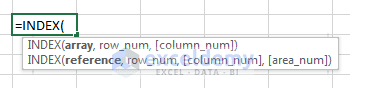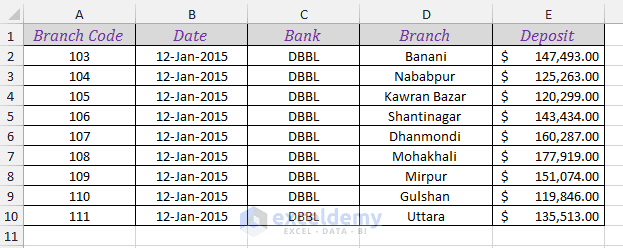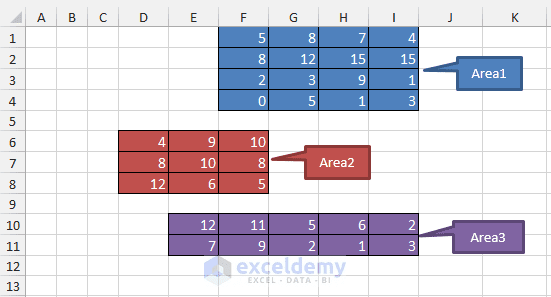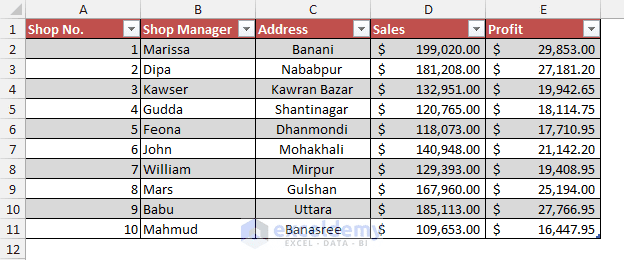Disclosure: This post may contain affiliate links, meaning when you click the links and make a purchase, we receive a commission.

# Index Function Excel [Examples, Make Dynamic Range, INDEX MATCH]

Do you know INDEX function is one of the top used 10 Excel functions? In this tutorial, you will get a complete idea of how INDEX function works in Excel individually and with other Excel functions. At first, I will cover the theory part and then I will show in how many ways you can use the INDEX function in Excel.

## What is INDEX Function in Excel

You will use INDEX function to return a value or a reference from a single range or from multiple ranges. You did read correctly, INDEX function returns also reference! Examples of references are A1, B1, E10, and so on. Very few Excel functions return a reference as the function output. INDEX function is one of them.

You will get INDEX function in two forms:

1. The array form: When you intend to return a value (or values) from a single range, you will use the array form of INDEX function.
2. And the reference form: when you intend to return a value (or values) from multiple ranges, you will use the reference form of INDEX function.

### Syntax of INDEX FunctionSyntax of INDEX function

Microsoft defines the INDEX function as: “Returns a value or reference of the cell at the intersection of a particular row and column, in a given range”.

In the image above, INDEX function shows two syntaxes: the first one is in array form and the second one is in reference form.

## How to Use the INDEX Function in Excel with Examples

You already know, you get INDEX function in two forms: in array form and in reference form. Let’s learn these two forms separately:

### Excel INDEX Function in Array Form:

Syntax of INDEX function in array form:

INDEX(array, row_num, [column_num])

The above syntax of INDEX function has the following arguments:

 Argument Required or not? Description array Required As this argument, you will pass a range of cells or an array constant. row_num Required As this argument, you will pass a number as the row_num. The INDEX function will return a value from the intersection of the row number and column number. If row_num is omitted, column_num is required. column_num Optional As this argument, you will pass a number as the column_num. The INDEX function will return a value from the intersection of the row number and column number.

NOTE:

1. If you use both the row_num and column_num arguments, INDEX function will return the value from the cell at the intersection of the row_num and column_num.
2. If you set row_num or column_num to 0 (zero), then you will get the whole column values or the whole row values as arrays respectively. You can insert those values into cells using Array Formula [we shall see it later].

NOTE:

Excel Web App has some limitations. One of them is: you cannot create array formulas in Excel Web App.

### Download Example Workbook & Practice INDEX Function

To follow along with this tutorial, first, download the working file(s) that I have used to create this tutorial.### Use of the INDEX Function in Array Form:

Let’s see how you can use the INDEX function in array form. Look at the following Excel worksheet. You get some deposits in different branches of DBBL bank on the same date. You will also get the code of the branches.Worksheet 1: Use of INDEX function in array form.

Based on the Excel worksheet above, the following INDEX formulas would return:

 Formula Explanation Result =INDEX(A1: E10, 5, 5) This formula will return the value at the intersection of the fifth row and fifth column in the range A1: E10. 143434 =INDEX(A1:E10, 3, 4) This formula will return the value at the intersection of the third row and fourth column in the range A1: E10. Nababpur =INDEX(A1:E10, 4, 0) This formula will return all the values of the fourth row in the range A1: E10. If you enter this formula into a cell normally, you might get #VALUE! type error. Insert this formula as an array formula into a cell, you will get the first value of the fourth row in the range A1: E10. #VALUE!Or105 =INDEX( A1: E10, 4) This formula will return a #REF! type error. When you pass a cell range with multiple columns as the array argument, you cannot omit the column_num argument. #REF! =INDEX({2, 5, 7, 8, 10, 3}, 4) This formula will return 8. When you use an array as the array argument, you can omit the column_num argument. 8 =INDEX(A1:E10, 4, 7) This formula will return #REF! error. As in the range A1: E10, there is no 7th column. #REF!

### Excel INDEX Function in Reference Form:

Syntax of the INDEX function in reference form:

INDEX(reference, row_num, [column_num], [area_num])

The above syntax of INDEX function has the following arguments:

 Argument Required or not? Description reference Required As this argument, you will pass one or more cell ranges. row_num Required As this argument, you will pass a number as the row_num. The INDEX function will return a reference from the intersection of the row number and column number. column_num Optional As this argument, you will pass a number as the column_num. The INDEX function will return a reference from the intersection of the row number and column number. area_num Optional As a reference argument, you can pass more than one cell range. The ranges are numbered as 1, 2, 3, … … Using area_num argument, you can select a range. If you use nothing then the first range will be selected.

### Use of the INDEX Function in Reference Form:

Let’s see how you can use the INDEX function in reference form. Look at the following Excel worksheet. Three cell ranges are named as Area1, Area2, and Area3. The ranges are colored in three different colors.Worksheet 2: Use of INDEX function in reference form.

Based on the Excel worksheet above, the following INDEX formulas would return:

 Formula Explanation Result =INDEX ((Area1, Area2, Area3), 2, 3) In the above formula, the area_num argument is omitted, so the INDEX function is using the first range, the Area1, and returning the value at the intersection of the second row and third column. The formula refers to cell H2 =INDEX ((Area1, Area2, Area3), 2, 3, 1) In the above formula, the area_num argument is 1, so the INDEX function is using the first range, the Area1, and returning the value at the intersection of the second row and third column. This formula also refers to cell H2 =INDEX ((Area1, Area2, Area3), 2, 3, 2) In the above formula, the area_num argument is 2. So, the INDEX function is using the second range, the Area2, and returning the value at the intersection of the second row and third column. This formula refers to cell F7

Master Excel Formulas & Functions in Just 3.5 Hours!

with my FREE COURSE at Udemy.

Excel Formulas and Functions with Excel Formulas Cheat Sheet!

## Some Important Uses of Excel INDEX Function

Now let’s see how INDEX function can be used in different situations:

### 1) Finding the nth Item from a List

Using INDEX function you can get easily the nth item from a list. The list may be a cell range with a single column or an array.

Range with Single Column: =INDEX (D1: D10, 5) = Shantinagar; see the Data of Worksheet 1.

Array: =INDEX ({7, 8, 2, 10, 45, 50, 15}, 4) = 10; 4th value of the array is 10

### 2) Get the Entire Row or Column from a Range

You can get the entire column or row from a cell range using the INDEX function. If row_num is 0 (zero) or empty, then the entire column will be selected and vice versa. See the table below:

 row_num column_num Result 0 or Empty 2 INDEX formula will select the entire 2nd column from the range. 5 0 or empty INDEX formula will select the entire 5th row from the range. Empty Empty Will show #REF! error.

Example 3 [Worksheet 1]: =INDEX(A1:E10, 4, 0) = will return the entire 4th row from the cell range A1: E10. At first select 5 blank horizontal cells in the worksheet, then insert this formula as the array formula into the cells.

Example 4 [Worksheet 1]: =INDEX(A1:E10, , 3) = will return the entire 3rd column from the cell range A1: E10. At first select 3 vertical blank cells in the worksheet, then insert this formula as the array formula into the cells.

Note:

When you use empty or 0 as the row_num or column_num arguments, entire column or row will be returned respectively.

### 3) Get the Value from the Intersection of Row and Column Number

You have already seen in action how to get the value from the intersection of the row and column numbers from a range.

### 4) INDEX Function Returns the Reference of Cells

When we write a formula like this: =INDEX (D1: D10, 5); this formula will return “Shantinagar”; [According to Worksheet 1 Data]. It seems that the formula is returning the value of cell D5. Actually, the formula returns the cell reference D5.

What’s the importance of this power of INDEX function?

Let’s check it with an example.

Say you write a formula like this: =SUM (A1: A100). You can also write this formula as =SUM (A1: INDEX(A1: A100, 100)). The last formula’s index part returns actually cell reference A100.

So  formula =SUM (A1: A100) and =SUM (A1: INDEX(A1: A100, 100)) are actually same.

Are you not impressed? You might think: why I shall write formula =SUM (A1: A100) as =SUM (A1: INDEX(A1: A100, 100))? The second version seems to be more complex. Proceed! You will find the true power of this feature.

### 5) Create Dynamic Range using the INDEX Function

You can create a dynamic range using the INDEX function. You can also create a dynamic range using the OFFSET function. But OFFSET function is a volatile function. On every change in your worksheet, the workbook will be refreshed. This will slow down your workbook.

Let’s see an example of using the OFFSET(…) function to create a dynamic range. Say you want to use the entire A column as your range.

This formula will create your dynamic range: =OFFSET ( A1, 0, 0, COUNTA (A: A), 1)

If you don’t understand the above formula then you have no idea how OFFSET and COUNTA functions work.

The INDEX function is a better choice to create dynamic ranges as the INDEX function is non-volatile. Non-volatile function means when you change something in your worksheet, the workbook is not updated immediately. So your workbook will not get slower. But when you will close and open the workbook, the non-volatile functions will get updated.

We can redesign the above OFFSET formula using INDEX function in this way: =A1: INDEX(A:A, COUNTA(A:A))

Assume that column A has values 45, 50, 55, 60, and 15 in cells A1, A2, A3, A4, and A5 respectively.

Let’s see the breakdown of the above formula:

1. COUNTA(A:A) will return 5 as the column has values in 5 cells. So the formula will be like =A1: INDEX(A:A, 5)
2. The INDEX part of the formula will now return the cell reference A5.
3. So the whole formula will be now like =A1: A5

## Use INDEX and Match Function instead of VLOOKUP Function

Do you know the VLOOKUP function has one limitation? It cannot go right. Look at the table below. The table is named as Sales_Table.Use INDEX & MATCH Functions Instead of VLOOKUP Function

If I say you to find out:

1. How many Sales are done by Shop Manager Feona? You can find out it using the VLOOKUP function. The formula will be: =VLOOKUP(“Feona”, \$B\$2: \$E\$10, 3, FALSE); if you don’t understand the formula, then take a look at how VLOOKUP function works.
2. But if I say you to find out who achieved the highest Sales? You cannot find out it using the VLOOKUP function, as you have to go from right to left. In this case, we use INDEX and MATCH functions.

### Using INDEX and MATCH functions to go from right to left in a table

To solve who achieved the highest sales, you can write a formula like this one for the above table: =INDEX(Sales_Table[Shop Manager], MATCH(MAX(Sales_Table[Sales]), Sales_Table[Sales], 0)); the name of the table is Sales_Table.

The above formula uses a total of 3 functions: INDEX, MATCH, and MAX functions. Let’s see the breakdown of the above formula:

1. MAX(Sales_Table[Sales]): This part of the formula finds out the highest Sales from the Sales column. The highest value is 199020. So the formula is now short to: =INDEX(Sales_Table[Shop Manager], MATCH(199020, Sales_Table[Sales], 0))
2. MATCH(199020, Sales_Table[Sales], 0): This part of the formula now finds out the position of the value 199020 in the Sales column. The position is 1. So the formula is now short to =INDEX(Sales_Table[Shop Manager], 1)
3. INDEX(Sales_Table[Shop Manager], 1): This part of the formula now finds out the 1st item from the list Shop Manager. It is Marissa.
4. So the entire formula returns the value, Marissa.

## Wrapping Up

So you see you can use INDEX function in many ways. I love the INDEX function very much. What about you? Do you know any INDEX function-related tricks that I add to my post? Please share in the comments box. I want to learn more about how you use the INDEX function in your works.

## Read More…

Hello!
Welcome to my Excel blog! It took me some time to be a fan of Excel. But now I am a die-hard fan of MS Excel. I learn new ways of doing things with Excel and share here. Not only how to guide on Excel, but you will get also topics on Finance, Statistics, Data Analysis, and BI. Stay tuned!

9 Comments
1. ReplyBaber Beg Jul 29, 2016 at 8:39 PM

Have tried the links to download the 1200+ macros examples e-book & 100+ excel functions cheat sheet. However, it keeps asking me to reload or re-register. Please if the file sizes are not too large can you forward to my email address.

• ReplyKawser Aug 1, 2016 at 10:32 AM

Baber,
I have sent you an email with instructions. Please check. I hope the email solves the problem.
Regards

2. ReplyAhmed Jul 30, 2016 at 6:39 PM

How about adding a link to save the content for later reference?
Ahmed Sheikh
ahmed_sheikh@hotmail.com

• ReplyKawser Aug 1, 2016 at 10:33 AM

You can do that Ahmed. Thank you.
Regards

3. ReplyWaleed Eltayeb Jul 31, 2016 at 4:26 PM

I have many data in columns A to E , I want to find all data that corresponding to a specific data from column A . This data from A may be exist three times or more.

4. ReplyWaleed Eltayeb Jul 31, 2016 at 4:30 PM

I have many data in columns A to E , I want to find all data from column E that corresponding to a specific data from column A. This data from A may be exist three times or more.

• ReplyKawser Aug 1, 2016 at 10:34 AM

Waleed,
Can you upload the working files of your problems? At least a sample file? If possible send an email to this address kawser@exceldemy.com

5. ReplyAug 24, 2016 at 6:45 AM

I am trying to use the index function to display a dollar amount listed in a table in the month that it will be billed for. I have multiple projects and when the formula is dragged down to the next project, the index gets off because the projects have different start dates. Is there a better way to have the index start at the first billing month other than copying the formula from the previous project to the first billing month of the next project?

6. Replyakshay thakker Sep 3, 2016 at 6:58 AM

Dear All:

I am looking for a way (without VBA) to create a dynamic array constant which has the value of {1,1,1;2,2,0;3,0,0} in column 3 and {1,1,1,1;2,2,2,0;3,3,0,0;4,0,0,0} in column 4.. and so on and so forth

This is where I have reached so far:
I was able to figure out that an array formula =CHOOSE(TRANSPOSE(A1:C1),{1,1,1},{2,2,0},{3,0,0}) where A1=1,B1=2,C1=3 gives me the solution and =A1*–(A1:A3<D1) gives me the value of {1,1,1}.. but when I try combining the above two into a single formula as =CHOOSE(TRANSPOSE(A1:C1),A1:C1*–(OFFSET(A1:C1,0,0,1,C1)<D1)).. i am returned the value of {1,2,3;#VALUE!,#VALUE!,#VALUE!;#VALUE!,#VALUE!,#VALUE!}….

I cant seem to figure out how to get the above formula to work or some other way to get the constant {1,1,1;2,2,0;3,0,0}.

Please do help.

Thanks
Akshay